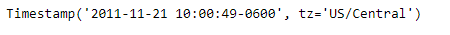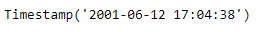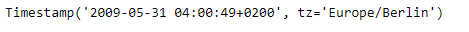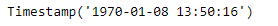Open in App
Not now

# Python | Pandas Timestamp.fromtimestamp

• Last Updated : 14 Jan, 2019

Python is a great language for doing data analysis, primarily because of the fantastic ecosystem of data-centric python packages. Pandas is one of those packages and makes importing and analyzing data much easier.

Pandas` Timestamp.fromtimestamp()` function return a Timestamp object when passed an integer which represents the timestamp value.

Syntax :Timestamp.fromtimestamp()

Parameters : integer

Return : Timestamp

Example #1: Use `Timestamp.fromtimestamp()` function to return a Timestamp object from the passed value.

 `# importing pandas as pd``import` `pandas as pd`` ` `# Create the Timestamp object``ts ``=` `pd.Timestamp(year ``=` `2011``,  month ``=` `11``, day ``=` `21``,``                  ``hour ``=` `10``, second ``=` `49``, tz ``=` `'US/Central'``)`` ` `# Print the Timestamp object``print``(ts)`

Output :Now we will use the `Timestamp.fromtimestamp()` function to return a Timestamp object from the passed value.

 `# convert to a Timestamp object``ts.fromtimestamp(``992345678``)`

Output :As we can see in the output, the `Timestamp.fromtimestamp()` function has returned a new Timestamp object constructed from the passed value.

Example #2: Use `Timestamp.fromtimestamp()` function to return a Timestamp object from the passed value.

 `# importing pandas as pd``import` `pandas as pd`` ` `# Create the Timestamp object``ts ``=` `pd.Timestamp(year ``=` `2009``, month ``=` `5``, day ``=` `31``,``                  ``hour ``=` `4``, second ``=` `49``, tz ``=` `'Europe/Berlin'``)`` ` `# Print the Timestamp object``print``(ts)`

Output :Now we will use the `Timestamp.fromtimestamp()` function to return a Timestamp object from the passed value.

 `# convert to a Timestamp object``ts.fromtimestamp(``634816``)`

Output :As we can see in the output, the `Timestamp.fromtimestamp()` function has returned a new Timestamp object constructed from the passed value.

My Personal Notes arrow_drop_up